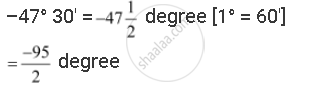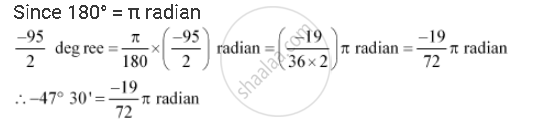CBSE (Arts) Class 11CBSE
Share

# Find the Radian Measures Corresponding to the Following Degree Measures:– 47° 30 - CBSE (Arts) Class 11 - Mathematics

#### Question

Find the radian measures corresponding to the following degree measures:

– 47° 30

#### Solution

–47° 30'Is there an error in this question or solution?

#### APPEARS IN

NCERT Solution for Mathematics Textbook for Class 11 (2018 to Current)
Chapter 3: Trigonometric Functions
Q: 1.2 | Page no. 54

#### Video TutorialsVIEW ALL 

Solution Find the Radian Measures Corresponding to the Following Degree Measures:– 47° 30 Concept: Concept of Angle.
S